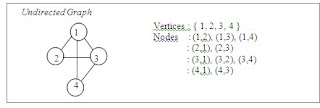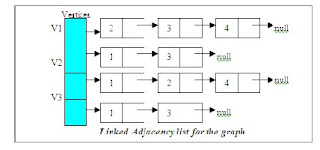/* related posts with thumb nails */

###Adjacency lists.:

Graphs can be represented as adjacency list that uses array of linked lists.

Consider the following graph. The graph has 4 vertices and it is undirected graph.4
 Vertices : { 1, 2, 3, 4 } Nodes : (1,2), (1,3), (1,4) : (2,1), (2,3) : (3,1), (3,2), (3,4) : (4,1), (4,3)
 Undirected Graph